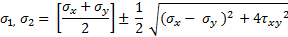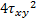# Principal stress?

HI everybody

I am in dire need of help. I have started a mechanical systems module as part of a distance learning foundation degree. The notes seem quite sparse to me and I can't seem to get to grips with it from the start. I have attached a photo of the tutorial questions but the are no workings to help me along can anyone give me some pointers. This is not an assignment it is just practice questions.

Any help would be appreciated.

#### Attachments

•image.jpg
87.2 KB · Views: 448

Chestermiller
Mentor
What is the specific difficulty you are encountering?

Chet

Hi Chet

Rather than me say everything I had better take it a step at a time, so here goes.

Question 1.

when it comes to determining the principal stress I haven't got any real clear guidance in the notes I have (or I just cant see it). can I assume that σy = 100MN/m2 and that σx = -45MN/m2. Then to get the principal stress I use:-now if I was right with my previous statement then I should be able to use this formula, but the stumbling block comes with "where do I getfrom."

Then once I have this I just use the formula with + then -.

Once again thanks

#### Attachments

Chestermiller
Mentor
It depends on what information you have available. The equation you presented assumes that you know the stress components with respect to the x and y coordinate directions.

If you know all the components of the stress, then you know τxy. So, no problem.

If you don't know all the components of the stress, then you might know all the components of the strain. If you know all the components of the strain, then you can use Hooke's law to get all the components of the stress.

If you don't know all the components of the strain, then you might know the three displacements. If you know the three displacements, then you can use the strain displacement equations to get all the components of the strain.

If you don't know any of these things, then you have to solve a deformational mechanics problem to determine them.

Chet

Ok all the questions I have seen online all specify the 3 values required but in the question I have been given I only had the 2 values. could you possibly tell me if in the question I am missing some information I require as I cant believe my tutor would make it this difficult at the start of the module. I believe I have the shear stress and the compressive stress can I get Txy from this.

Chestermiller
Mentor
Ok all the questions I have seen online all specify the 3 values required but in the question I have been given I only had the 2 values. could you possibly tell me if in the question I am missing some information I require as I cant believe my tutor would make it this difficult at the start of the module. I believe I have the shear stress and the compressive stress can I get Txy from this.

The shear stress is Txy.

Chet

ok i'm starting to see where things fit in. if I may clarify then σy is compressive stress 100MN/m2 and sheer stress is τxy -45MN/m2, but what value is σx. the question states "on a plane perpendicular to the one carrying the compressive stress only complementary shear exists" this I am assuming is σx but have no idea what the value is. once I know this I think I may have cracked it.

Chestermiller
Mentor
ok i'm starting to see where things fit in. if I may clarify then σy is compressive stress 100MN/m2 and sheer stress is τxy -45MN/m2, but what value is σx. the question states "on a plane perpendicular to the one carrying the compressive stress only complementary shear exists" this I am assuming is σx but have no idea what the value is. once I know this I think I may have cracked it.
I'm having trouble understanding what you are asking. Let's work a specific problem and see how it plays out. But the photo you sent is unreadable(by me), so please type out the question. Thanks.

Chet

ah sorry I didn't know it was unreadable. here goes.

An axle of a go kart is loaded in such a way that on a surface plane aligned circumferentially a direct compressive stress of 100MN/m2 is applied. In association with the compressive stress is a shear stress applied from the driving torque equivalent to -45MN/m2. On a plane perpendicular to the one carrying the compressive stress only complementary shear exits.

a) Sketch a portion of the axle highlighting the stress system.
b) Determine the principal stresses.
c) Determine the Maximum Stress.
d) Sketch a Mohrs stress circle for the system and compare the estimate of the orientation of the principal planes from the circle with that obtained exactly.

I have the answers which are (17.3, -117.3 & 67.3MN/m2) but I do not know how to obtain them.

Hope this helps explain.

Chestermiller
Mentor
Ah. OK. I think I understand the problem.

The axle is a cylinder, so we're going to be using a cylindrical polar coordinate system with the axis of the cylinder being the z direction.

Do you use the criterion that compressive stresses are positive, or do you use the criterion that tensile stresses are positive?

In this problem, you are looking at the state of stress at the surface of the cylinder, r = R. According to the problem description, the only non-zero components of stress at r = R are σzz, σθθ, and σ. First you look at a plane perpendicular to the axis of the cylinder (i.e., "circumferentially aligned"), and examine the normal and shear stresses on this plane at r = R. Which of the three stresses that I listed are these? What are the values of these stresses?

To be continued.

Chet

I may be barking up the wrong tree here but if we are using the z axis then isn't this a 3D analysis. I am currently only doing 2D analysis so do the Compressive stress of 100 MN/m2 and the shear stress of -45MN/m2 only apply to the x and y axis with 100MN/m2 being on the y axis. The notes i have been given by my tutor seem very inadequate to me and dont lead into the questions very well.

Chestermiller
Mentor
This is definitely 2D. Not all problems use xy coordinates. Some use θz coordinates. Are you able to visualize these stresses on the surface of the cylinder?

Chet

I believe I do. Is theta going around the surface with z going through the axle. I had a look online and think this is what I am seeing.

Chestermiller
Mentor
I believe I do. Is theta going around the surface with z going through the axle. I had a look online and think this is what I am seeing.
Yes. Yes. Yes.

So now what are the values of the zz, θθ, and zθ stresses?

Chet

This is so frustrating I am only seeing the 2 values in the question so I'm assuming one value is 0. Is zz 100MN/m2 and theta theta -45MN/m2 and z theta 0. I'm pulling my hair out trying to get my head round This.

Chestermiller
Mentor
This is so frustrating I am only seeing the 2 values in the question so I'm assuming one value is 0. Is zz 100MN/m2 and theta theta -45MN/m2 and z theta 0. I'm pulling my hair out trying to get my head round This.
The zz is -100 (compressive).
The zθ is -45.
The θθ is 0.

Chet

Damn I thought I had it then. So all I need to do know is find the right formula to input these values into.

Chestermiller
Mentor
Damn I thought I had it then. So all I need to do know is find the right formula to input these values into.
You should try to figure out how these stresses are related to your original problem description. As far as the equation for the principal stresses are concerned, you already had that in post #3.

So I have been trying to look at this again today. I have the principal stress formula but as we are not using x and y I take it we are using the zz, theta z & theta theta values. I'm sorry but I just can't see how these values factor into the formula.

Chestermiller
Mentor
Replace xx by zz, xy by zθ, and yy by θθ.

Chet